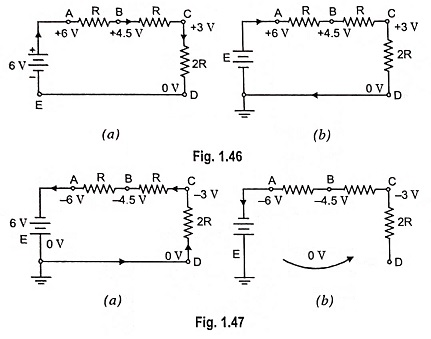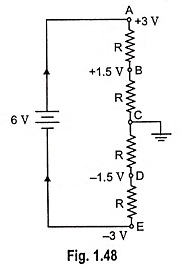## Zero Reference Level in Electronics Engineering:

Zero Reference Level – In order to avoid errors in the measurement of different voltages in an electronic circuit, it is imperative to select some common point, known as reference, zero potential or datum point and measure all circuit voltages (whether positive or negative) with respect to this point.

The point to be selected as reference point can be any point in the circuit and need not be necessarily at zero volt. In Fig. 1.46, negative terminal of battery i.e., point E is taken as reference point and voltages of other points in the circuit are mentioned with reference to this point.For instance, voltages of points A, B and C, with respect to negative battery terminal (zero reference level) are respectively +6 V, +4.5 V and +3 V respectively and voltage of point D with respect to reference point E is zero because it is directly connected to E. The negative battery terminal can be grounded as depicted by 3 short lines in Fig. 1.46 (b).

Even if positive terminal of battery is made reference point [Fig. 1.47 (a)] there would be hardly any difference in voltage measurement. The only difference is that now all circuit voltages would be negative with reference to zero potential or ground point, but magnitude would remain unchanged. Direction of flow of current, however, would be reversed.

The noteworthy point is that we are primarily concerned with the magnitude of voltage of any point with respect to reference point and that does not change with the change of reference point. Figure 1.47 (b) depicts another way of showing ground in circuit schematic diagram.In Fig. 1.48, four equal resistors are connected in series across a 6 V battery. The point C has been grounded i.e., fixed as zero reference level for voltage measurements. It is seen that point B is 1.5 V above point C (reference point) i.e., it is positive with respect to point C whereas point D is 1.5 V below point C i.e., it is negative with respect to point C. Similarly, point A is 3 V above point C and point E is 3 V below point C.

Another noteworthy point is that conventional current always flows from a point at higher potential to one at lower potential. Since A is at higher potential as compared to point C, current flows from point A to C and for the same reasons, current flow is from C to E.

Scroll to Top